# Functional derivative

Volterra derivative

One of the first concepts of a derivative in an infinite-dimensional space. Let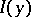be some functional of a continuous function of one variable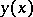; letbe some interior point of the segment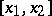; let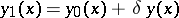, where the variationis different from zero in a small neighbourhoodof; and let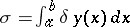. The limit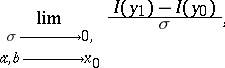assuming that it exists, is called the functional derivative ofand is denoted by. For example, for the simplest functional of the classical calculus of variations,the functional derivative has the formthat is, it is the left-hand side of the Euler equation, which is a necessary condition for a minimum of.

In theoretical questions the concept of a functional derivative has only historical interest, and in practice has been supplanted by the concepts of the Gâteaux derivative and the Fréchet derivative. But the concept of a functional derivative has been applied with success in numerical methods of the classical calculus of variations (see Variational calculus, numerical methods of).

The existence of the functional derivative ofat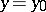andapparently means that the Fréchet derivativeofat, which is a continuous linear form on the space of admissible infinitesimal variations, is of the form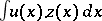for some continuous function, so that it can be continuously extended to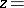the-function at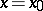. In the example this happens only if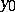is twice continuously differentiable.